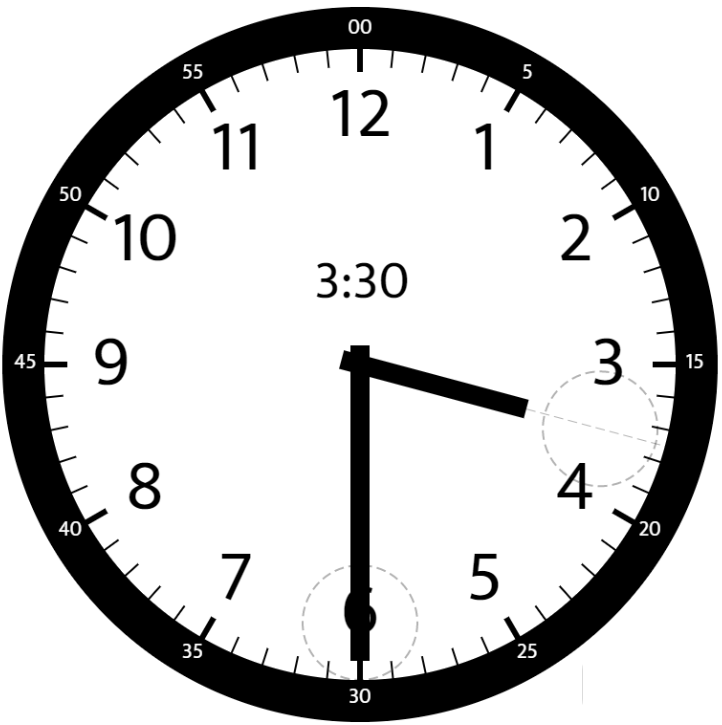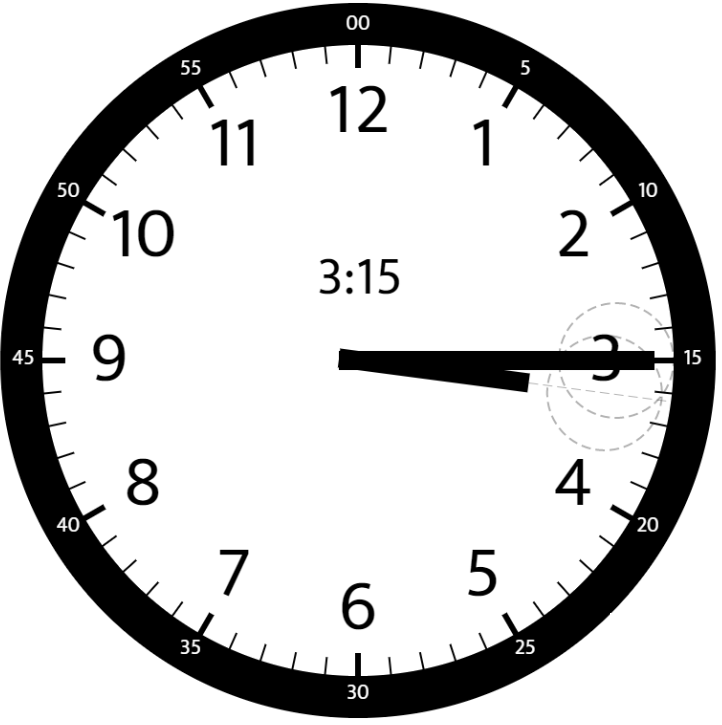##### Welcome to Subscribe On Youtube

Formatted question description: https://leetcode.ca/all/1344.html

# 1344. Angle Between Hands of a Clock (Medium)

Given two numbers, hour and minutes. Return the smaller angle (in sexagesimal units) formed between the hour and the minute hand.

Example 1:Input: hour = 12, minutes = 30
Output: 165


Example 2:Input: hour = 3, minutes = 30
Output: 75


Example 3:Input: hour = 3, minutes = 15
Output: 7.5


Example 4:

Input: hour = 4, minutes = 50
Output: 155


Example 5:

Input: hour = 12, minutes = 0
Output: 0


Constraints:

• 1 <= hour <= 12
• 0 <= minutes <= 59
• Answers within 10^-5 of the actual value will be accepted as correct.

Related Topics:
Math

## Solution 1.

• class Solution {
public double angleClock(int hour, int minutes) {
double hoursDegree = hour * 30 + minutes * 30.0 / 60;
int minutesDegree = minutes * 6;
double angle = Math.abs(minutesDegree - hoursDegree);
if (angle > 180)
angle = 360 - angle;
return angle;
}
}

• // OJ: https://leetcode.com/problems/angle-between-hands-of-a-clock/
// Time: O(1)
// Space: O(1)
class Solution {
public:
double angleClock(int h, int m) {
double a = h * 30 + m * 0.5, b = m * 6, d = abs(a - b);
return min(d, 360 - d);
}
};